##### The Japanese Maple specialist
Direct order Contact Help / Services Newsletter# Shelf to displaySearch

Japanese MaplesYoung trees Acer seeds and more Bonsai soil and fertiliserVarieties introduced into EuropeHostasWind bells and chimesSaxifragesSpecial offer

Packing and shipping charges

# Mikawa yatsubusa

› Japanese maples › Acer palmatum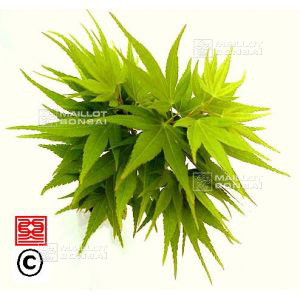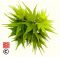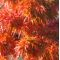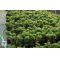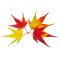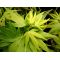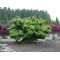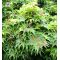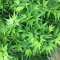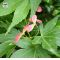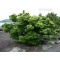ref. : 667

Choose the right size
Pot size

This item is temporarily unavailable

###### Description

Green spring foliage to stretch between short knots, in dark green summer and in splendid fall: orange late red. Slow growth. Form a bush in the gracious port, as aging the trunk becomes sinuous. Please note that the specimens in 1.5 liter pots do not measure more than 25 cm in height. 30-40 for 3 liter pot and 40 to 60 for 4 liter pot.

#liter 3.5 #yatsubusa 2.9 #palmatum 2.8 #mikawa 2.6 #green 2.5 #acer 2.4 #specimens 1.9 #splendid 1.8 #gracious 1.8 #becomes 1.7

###### Technical description
 Species Acer palmatum same species items Variety Mikawa yatsubusa Leaf colour Green leaves same colour items Leaf shape Palmated same shaped items Adult height Less than 2 meters same height items Port Tabular same port items Expositions Sun same exposition items

Formule
(( ROUND((CHAR_LENGTH(b.article_nom)-CHAR_LENGTH(REPLACE(b.article_nom, 'liter', '')))/LENGTH('liter')) + ROUND((CHAR_LENGTH(b.article_description)-CHAR_LENGTH(REPLACE(b.article_description, 'liter', '')))/LENGTH('liter')) ) * 3.5) + (( ROUND((CHAR_LENGTH(b.article_nom)-CHAR_LENGTH(REPLACE(b.article_nom, 'yatsubusa', '')))/LENGTH('yatsubusa')) + ROUND((CHAR_LENGTH(b.article_description)-CHAR_LENGTH(REPLACE(b.article_description, 'yatsubusa', '')))/LENGTH('yatsubusa')) ) * 2.9) + (( ROUND((CHAR_LENGTH(b.article_nom)-CHAR_LENGTH(REPLACE(b.article_nom, 'mikawa', '')))/LENGTH('mikawa')) + ROUND((CHAR_LENGTH(b.article_description)-CHAR_LENGTH(REPLACE(b.article_description, 'mikawa', '')))/LENGTH('mikawa')) ) * 2.6) + (( ROUND((CHAR_LENGTH(b.article_nom)-CHAR_LENGTH(REPLACE(b.article_nom, 'green', '')))/LENGTH('green')) + ROUND((CHAR_LENGTH(b.article_description)-CHAR_LENGTH(REPLACE(b.article_description, 'green', '')))/LENGTH('green')) ) * 2.5) + (( ROUND((CHAR_LENGTH(b.article_nom)-CHAR_LENGTH(REPLACE(b.article_nom, 'specimens', '')))/LENGTH('specimens')) + ROUND((CHAR_LENGTH(b.article_description)-CHAR_LENGTH(REPLACE(b.article_description, 'specimens', '')))/LENGTH('specimens')) ) * 1.9) + (( ROUND((CHAR_LENGTH(b.article_nom)-CHAR_LENGTH(REPLACE(b.article_nom, 'gracious', '')))/LENGTH('gracious')) + ROUND((CHAR_LENGTH(b.article_description)-CHAR_LENGTH(REPLACE(b.article_description, 'gracious', '')))/LENGTH('gracious')) ) * 1.8) + (( ROUND((CHAR_LENGTH(b.article_nom)-CHAR_LENGTH(REPLACE(b.article_nom, 'splendid', '')))/LENGTH('splendid')) + ROUND((CHAR_LENGTH(b.article_description)-CHAR_LENGTH(REPLACE(b.article_description, 'splendid', '')))/LENGTH('splendid')) ) * 1.8) + (( ROUND((CHAR_LENGTH(b.article_nom)-CHAR_LENGTH(REPLACE(b.article_nom, 'between', '')))/LENGTH('between')) + ROUND((CHAR_LENGTH(b.article_description)-CHAR_LENGTH(REPLACE(b.article_description, 'between', '')))/LENGTH('between')) ) * 1.7) + (( ROUND((CHAR_LENGTH(b.article_nom)-CHAR_LENGTH(REPLACE(b.article_nom, 'sinuous', '')))/LENGTH('sinuous')) + ROUND((CHAR_LENGTH(b.article_description)-CHAR_LENGTH(REPLACE(b.article_description, 'sinuous', '')))/LENGTH('sinuous')) ) * 1.7) + (( ROUND((CHAR_LENGTH(b.article_nom)-CHAR_LENGTH(REPLACE(b.article_nom, 'stretch', '')))/LENGTH('stretch')) + ROUND((CHAR_LENGTH(b.article_description)-CHAR_LENGTH(REPLACE(b.article_description, 'stretch', '')))/LENGTH('stretch')) ) * 1.7)

## Secure payment## Delivery

Our logistic partners :04 74 55 23 48
Pépinière MAILLOT-BONSAÏ
Le Bois Frazy
01990 RELEVANT - FRANCE
on appointment